# Generic Spatial Data Types Support

Applies to: Denodo 8.0 , Denodo 7.0 , Denodo 6.0
Tags:

You can translate the document:

# Introduction

Currently, Virtual DataPort does not include native support for spatial data types. However, this does not mean that we cannot read its content or operate with it.

This document explains how to deal with spatial data from any system that follows the Simple Features standard  in Virtual DataPort, using custom functions. Specifically, this solution has been successfully tested with IBM DB2, Microsoft SQL Server and Oracle.

# Import Spatial Data

The Simple Features standard defines a model to represent and store spatial data. This model understands spatial data as vector geometries (point, lines, polygons…) of two dimensions. In order to interpret these geometries we need to know the spatial reference system in which their coordinates are expressed.

The Simple Features standard also defines an API to deal with spatial data. This API includes methods to obtain a formal textual representation of the geometry (Well-Known Text – WKT), a formal binary representation of the geometry (Well-Known Binary – WKB) and its spatial reference system (Spatial Reference System ID – SRID).

To import spatial data from Virtual DataPort, create a base view from a query that uses the Simple Features API to obtain these components:

• A formal representation of the geometry
• The SRID

The Figure 1 shows a query that retrieves both formal representations and the SRID from IBM DB2.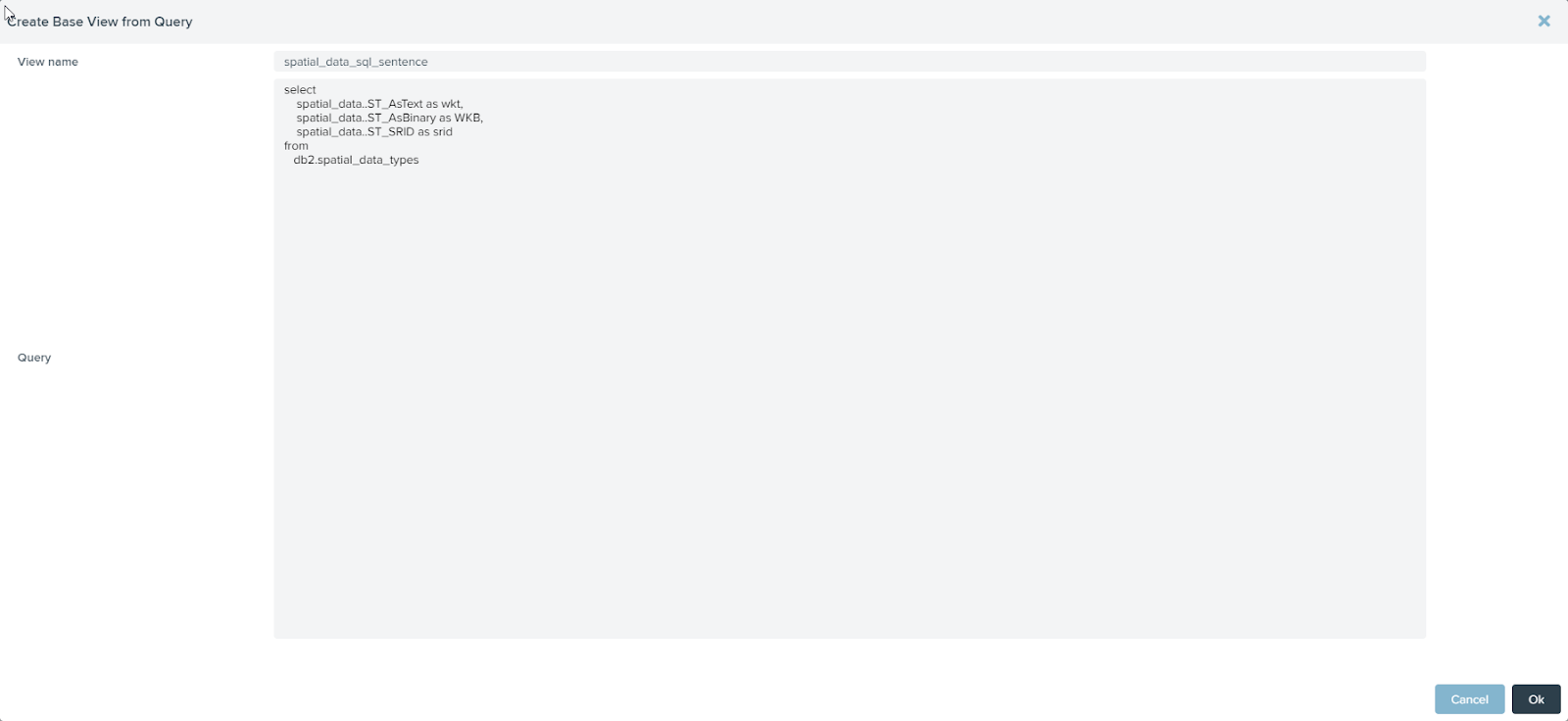Figure 1 Query that obtains the WKT, the WKB and the SRID of a geometry from IBM DB2

The Figure 2 shows the same query, for Microsoft SQL Server.

Note that the spatial_data field in the data source is of geometry type, a data type that Virtual DataPort does not support. The purpose of using a query to create a base view is to convert this geometry type into data types that Virtual DataPort can handle. As we can see in Figure 3, the base view just created is defined in terms of text, blob and int data types.

The Figure 4 shows the result of executing the view.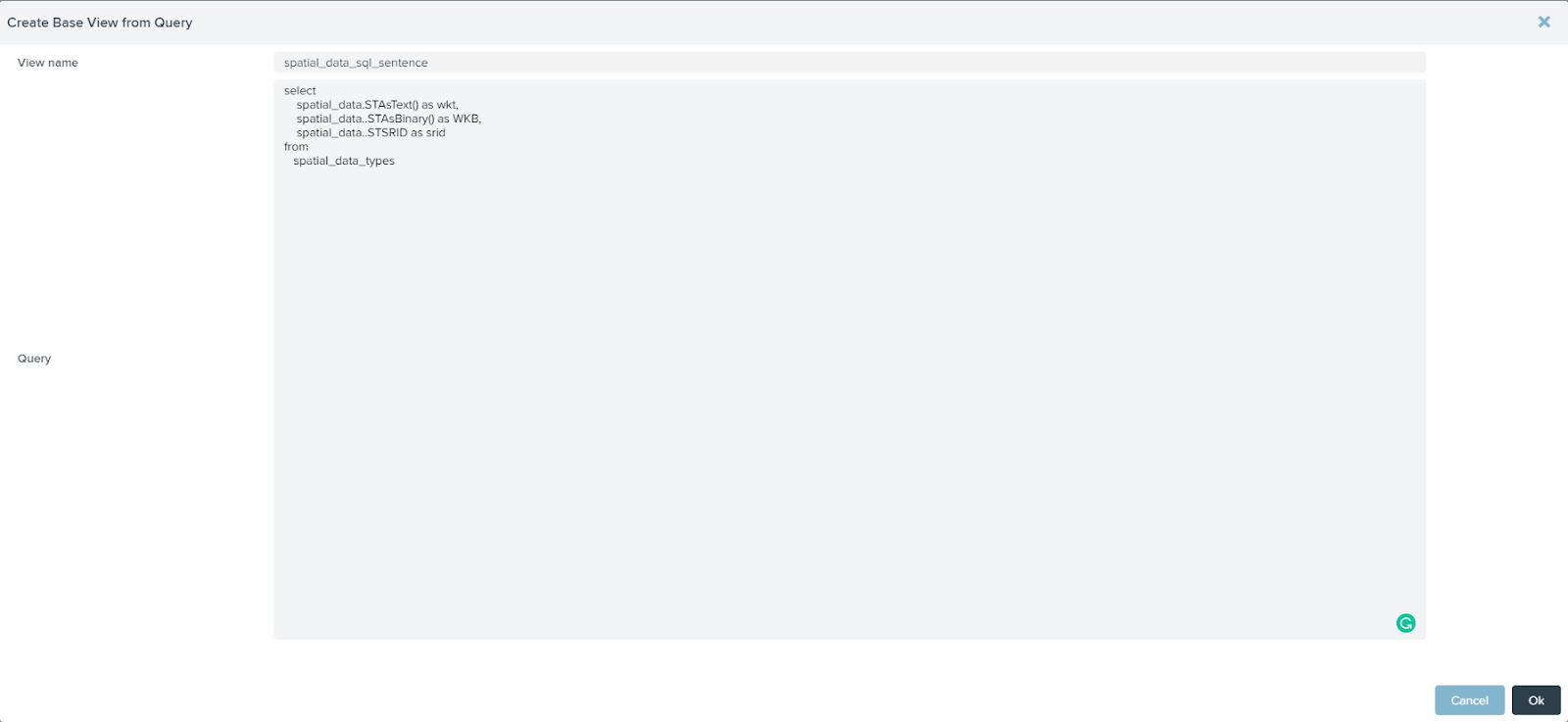Figure 2 Query that obtains the WKT, the WKB and the SRID of a geometry from Microsoft SQL Server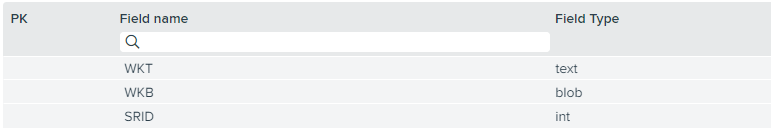Figure 3 View that obtains the WKT, the WKB and the SRID of a geometry from Virtual DataPort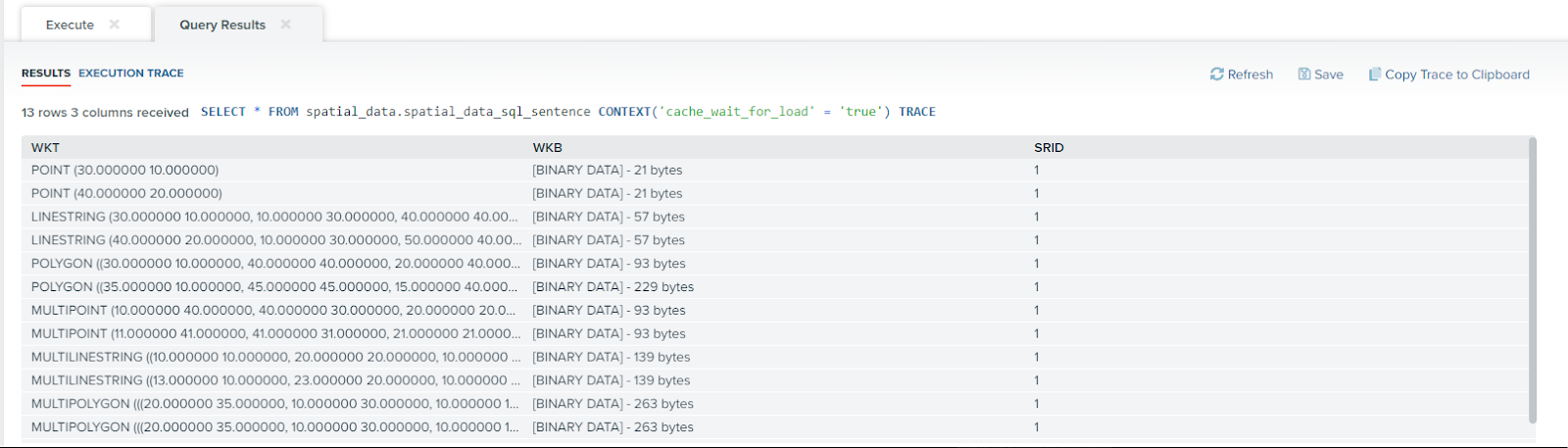Figure 4 Execution of the view that obtains the WKT, the WKB and the SRID of a geometry from Virtual DataPort

## Import spatial data from Introspection dialog

If we do not need to know the value of the SRID, VDP allows us to create the base view from the introspection dialog (see Figure 5).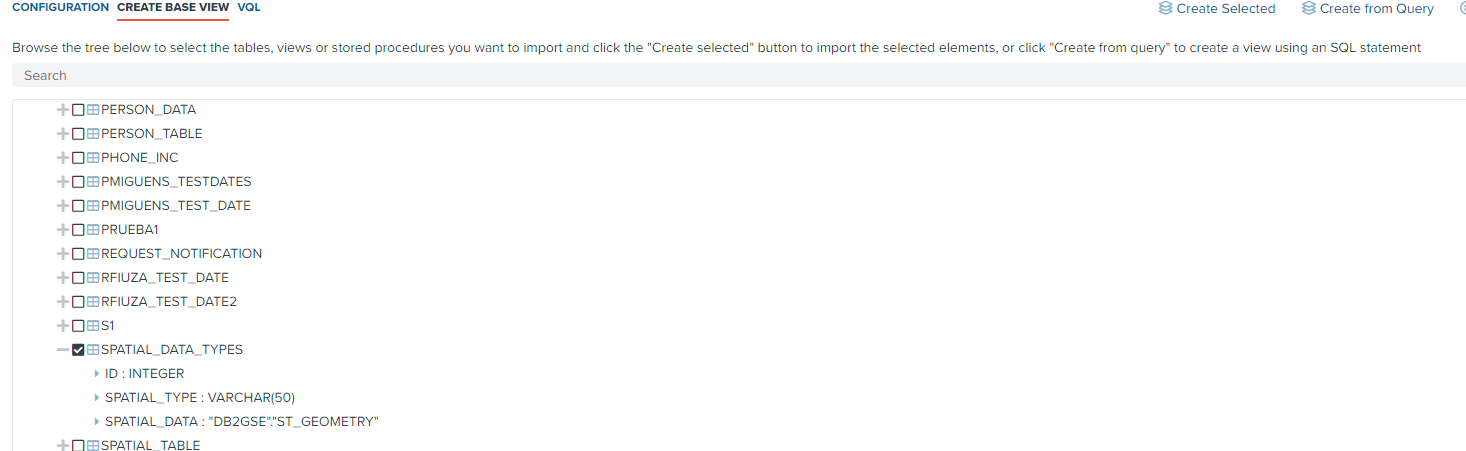Figure 5 Introspection of a table with spatial data in IBM DB2

The Figure 6 shows how in this case, Virtual DataPort interprets the spatial data type as text.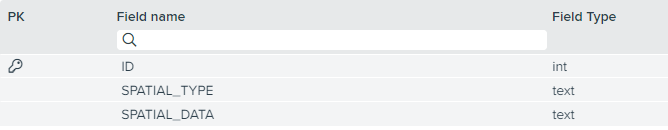Figure 6 View with spatial data from IBM DB2 interpreted as text in Virtual DataPort

As we can see in Figure 7, if we execute the view created from the introspection dialog, Virtual DataPort will provide the Well-Known Text of the spatial data as a result of interpreting this field as text.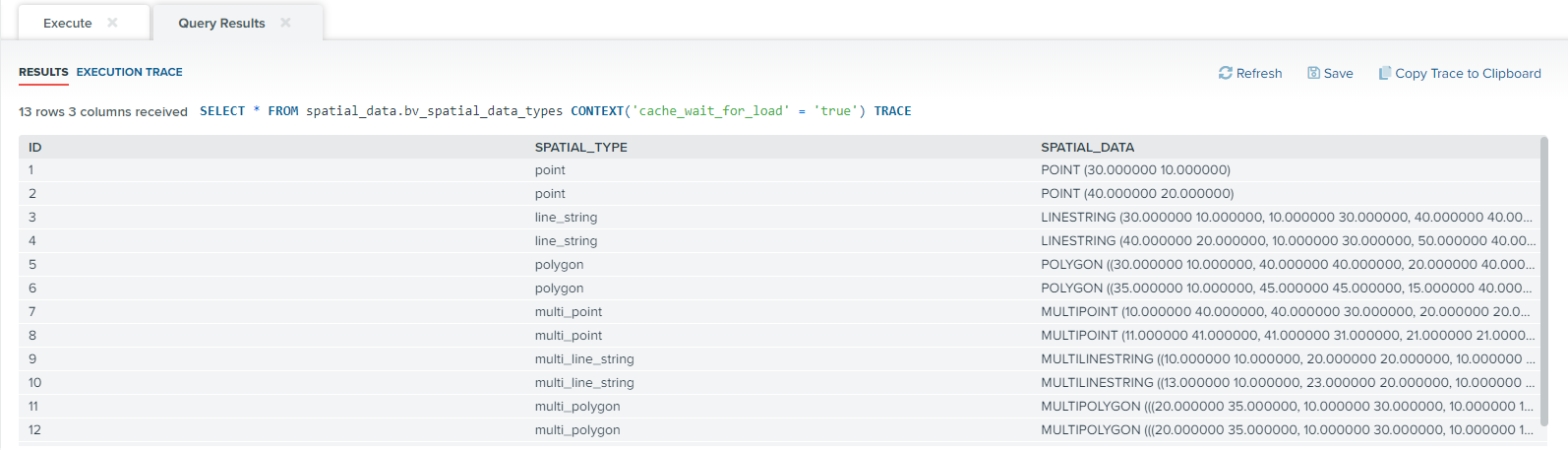Figure 7 Execution of the view with spatial data from IBM DB2 interpreted as text in Virtual DataPort

# Operate with spatial data

Virtual DataPort currently does not include any function to deal with spatial data. However, the functions set of Virtual DataPort can be extended by developing custom functions that augment the operations it can handle . In order to operate with spatial data, we can develop a custom function that reads a geometry expressed as WKT or WKB and performs whatever spatial operation on it.

Since spatial operations can be quite complex, we strongly recommend using a software library that provides the geometric operation we need. For example, the JTS Topology Suite is an open source Java library that implements the spatial functions specified in the Simple Features standard. It is freely available to download from http://sourceforge.net/projects/jts-topo-suite/.

As an example, Figure 8 shows a custom function that uses the JTS Topology Suite. The custom function is called st_distance and calculates the minimum distance between two geometries expressed as WKB.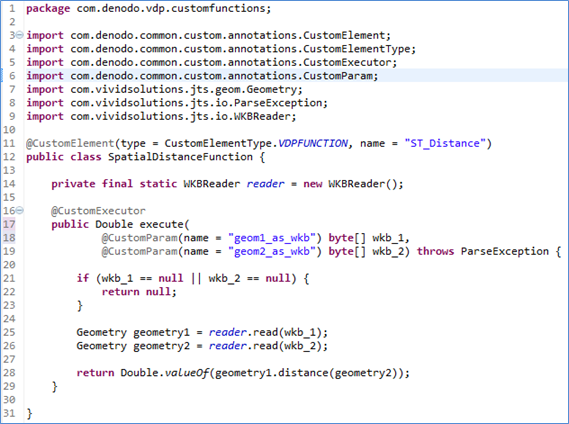Figure 8 Custom function that implements the spatial function distance using the JTS Topology Suite.

The Denodo Xtrafuncs for VDP library  includes the custom function st_geom_to_struct, which is another example of operation that can be performed on spatial data from Virtual DataPort. This function receives a WKT or a WKB as input, parses it  and transforms it in a structural representation of the geometry in terms of registers and arrays. The return type of the function has these fields (see Figure 9):

• type: the geometry type (Point, Linestring, Polygon…).
• bounding_box: the coordinates of the minimum rectangle that contains the geometry.
• point: the coordinates x and y of the point, if the type field states that the geometry is a point.
• linestring: an array with the coordinates of the points that form the linestring, if the type field states that the geometry is a linestring.
• polygon: the exterior linestring (a.k.a. shell) and an array with the holes in the polygon, if the type field states that the geometry is a polygon.
• multipoint: an array with all its points, if the type field states that the geometry is a multipoint.
• multilinestring: an array with all its linestrings, if the type field states that the geometry is a multilinestring.
• multipolygon: an array with all its polygons, if the type field states that the geometry is a multipolygon.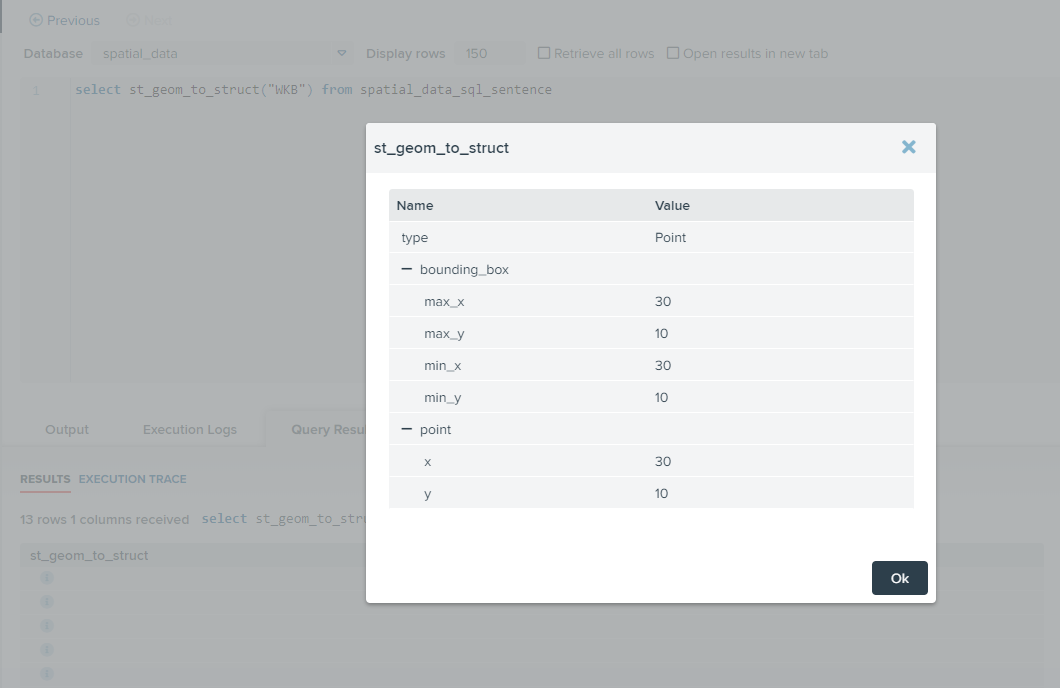Figure 9 Return type of the custom function st_geom_to_struct for WKB

# Use Case: Get the location of a picture

Let us assume a system of geo-tagging pictures where the pictures are displayed in a map according to where they were taken.

This example explains how to obtain the coordinates of the pictures by reading the spatial data as explained before.

First, we import the geo_picture table from the data source with the query shown in Figure 10. In this table, the location field stores the point in the space where the picture was taken.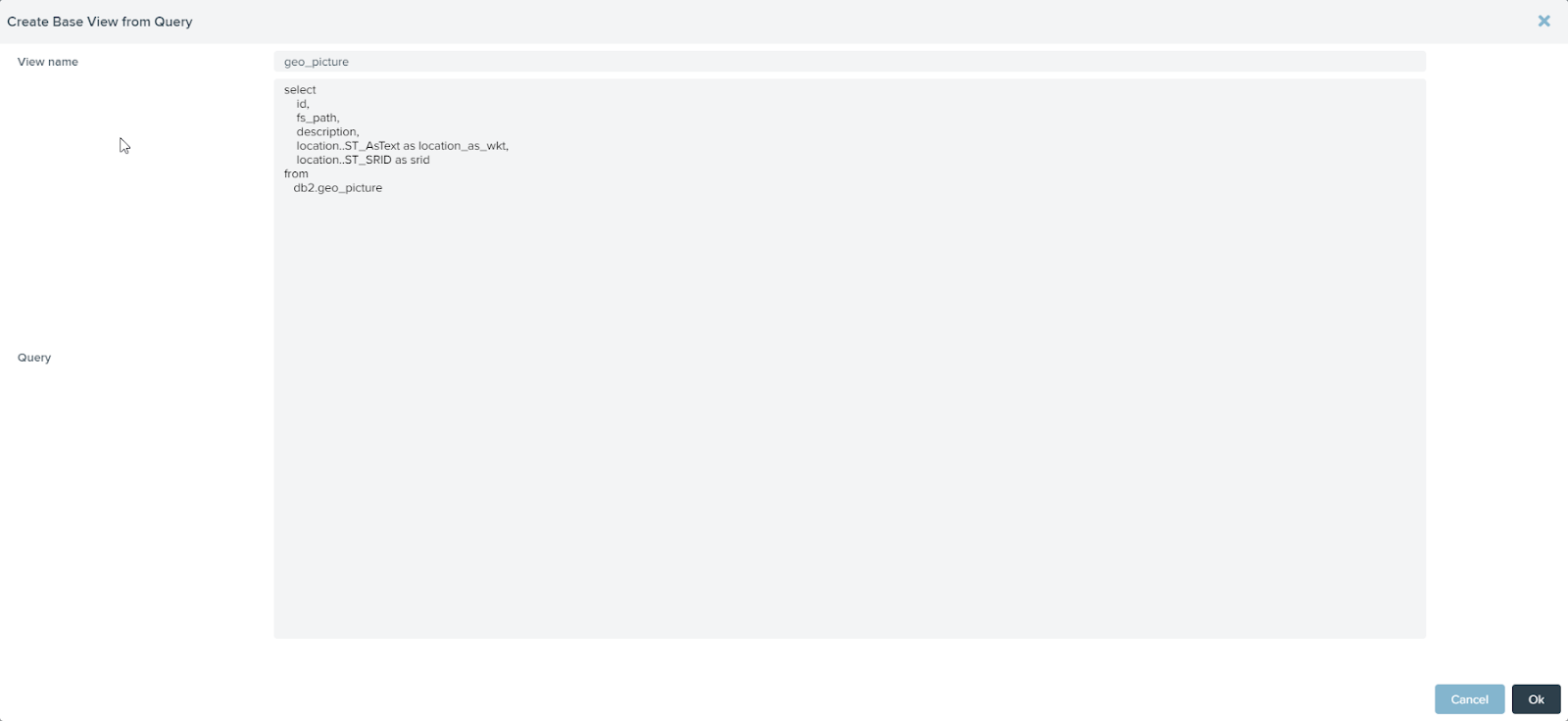Figure 10 Query to create a view of a table with geo-tagged pictures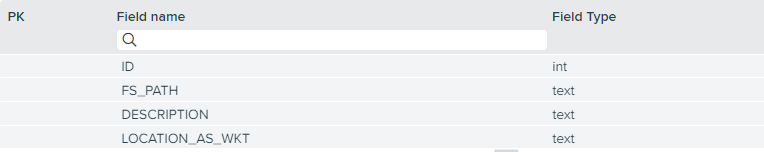Figure 11 View with information about the geo-tagged pictures

Next, we create a projection view over bv_geo_picture where the field location_as_wkt is replaced by a call to the st_geom_to_struct custom function (see Figure 12).Figure 12 New field that uses the geom_to_stuct custom function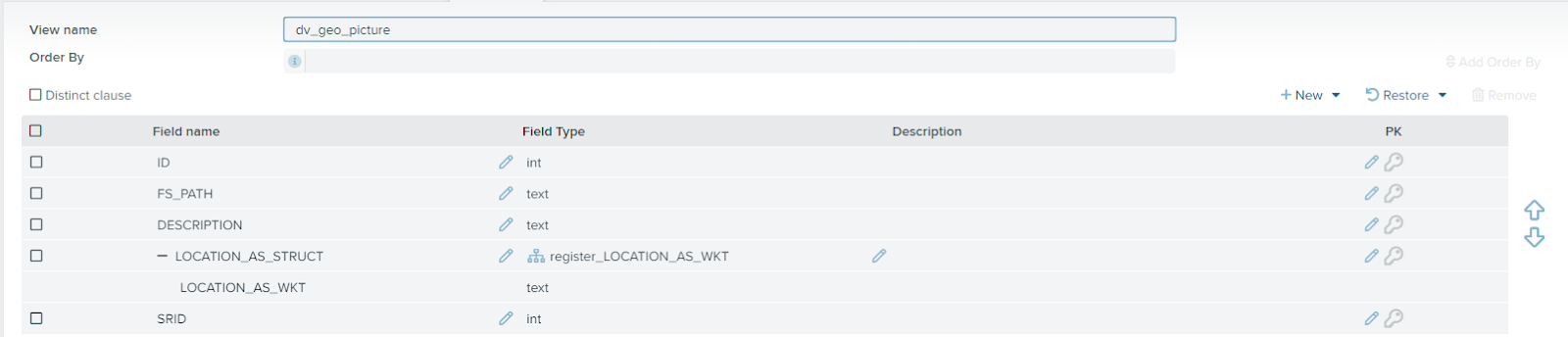Figure 13 View with information about the geo-tagged pictures that uses the geom_to_stuct custom function

To extract the coordinates where the pictures were taken, use the query of Figure 14.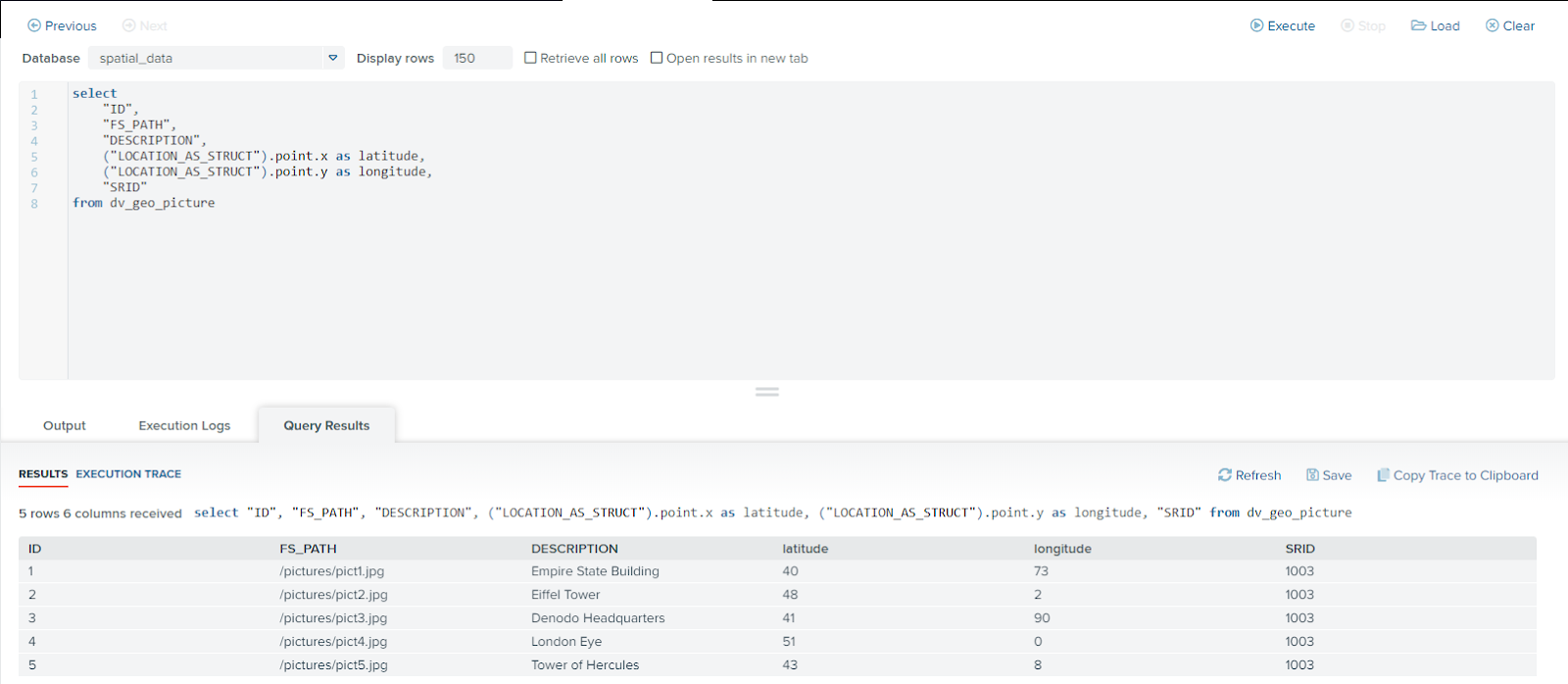Figure 14 Query that obtains the coordinates of geo-tagged pictures

# Use Case: Get the n-nearest pictures

One of the most common queries in a spatial system is to calculate the nearest neighbors of a geometry.

The Figure 15 shows how to create a base view from a query that obtains the nearest neighbors of a picture, ordered by the distance between pictures. This query depends on two parameters: the id of the target picture and the number of neighbors.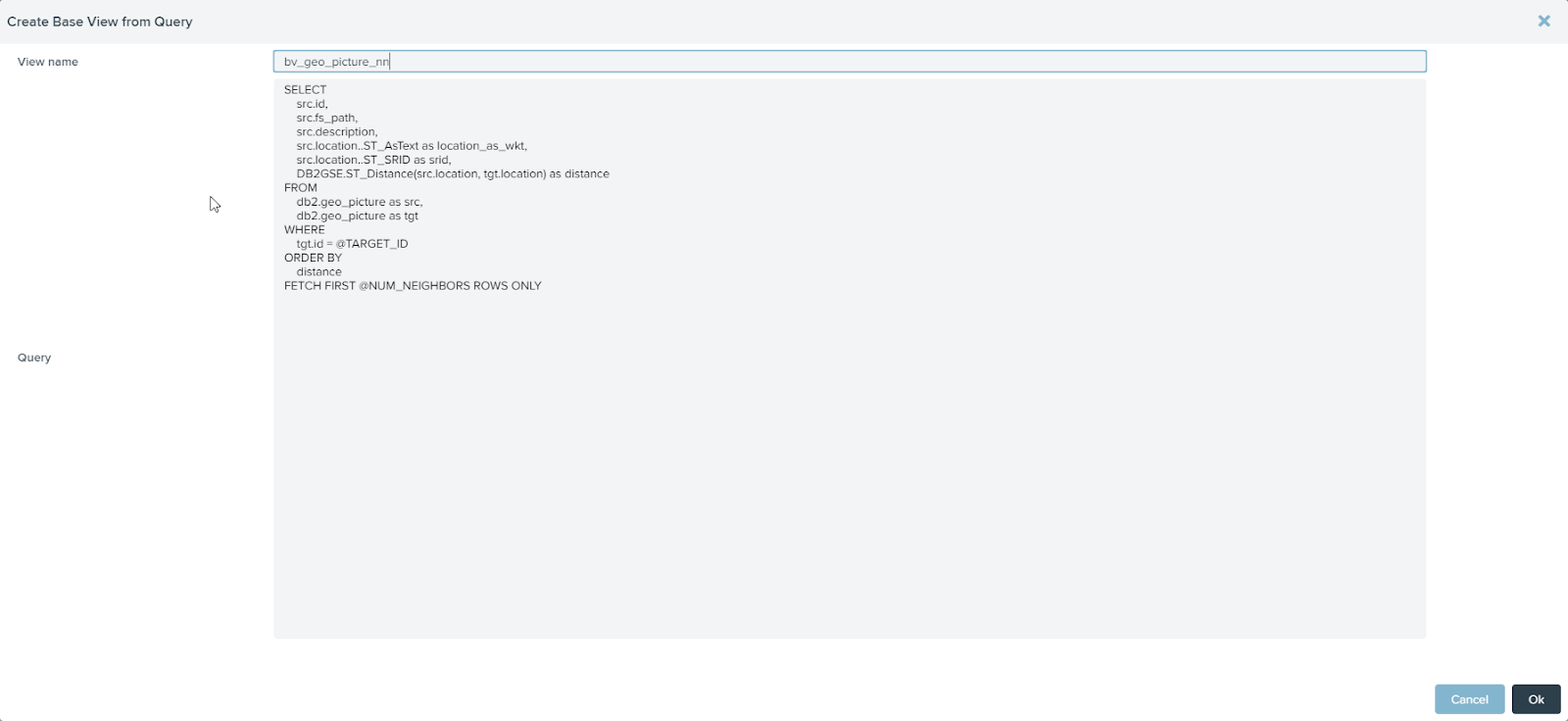Figure 15 Query that calculates the nearest pictures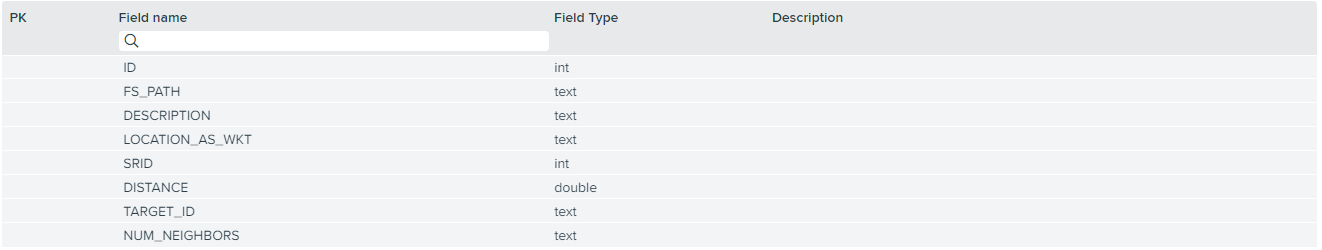Figure 16 View that obtains the nearest pictures

As in the previous example, we create a projection view over the view geo_picture_nn that uses the st_geom_to_struct custom function (see Figure 17).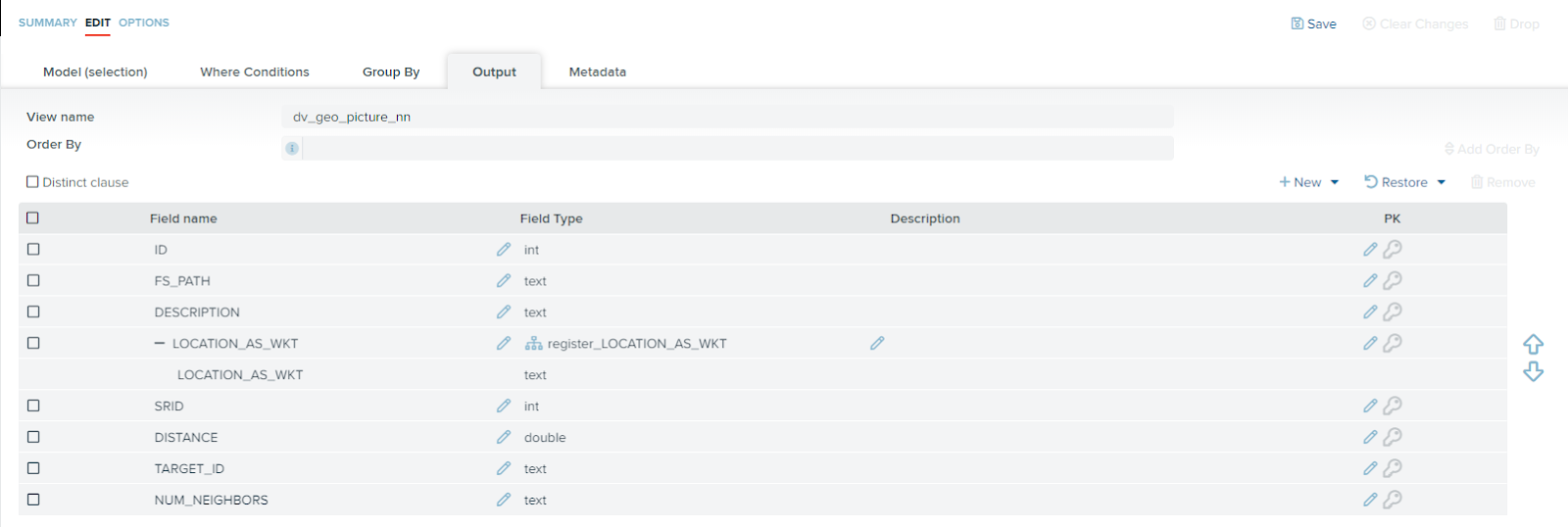Figure 17 View that obtains the nearest pictures and uses the geom_to_stuct custom function

The Figure 18 shows a query in Virtual DataPort that obtains the three nearest pictures to the picture with id 4.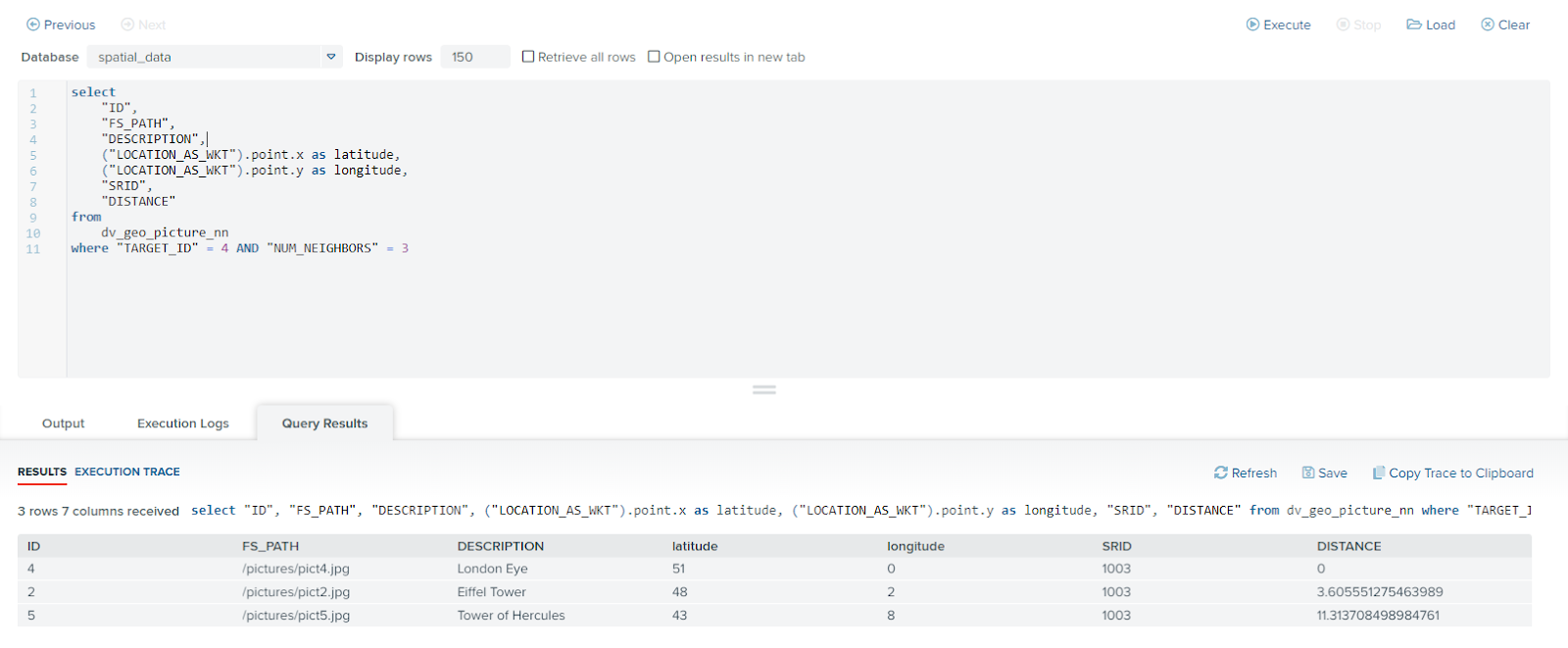Figure 18 Query that obtains the nearest pictures in Virtual DataPort

1. A Microsoft SQL Server database with the points of interest of a city.
2. An IBM DB2 database with the routes of the bus lines of that city.

We assume that the spatial data in both data sources are expressed in the same spatial reference system. Our aim is to obtain the closest bus line for each point of interest in the city. Let’s see how to achieve it using Denodo.

First of all, we have to import the spatial data in Virtual DataPort using a query for each one of the data sources. The Figure 19 and the Figure 20 show the queries we have used to import the points of interest and the routes, respectively. Note that the fields location and route are geometries which are imported as WKB.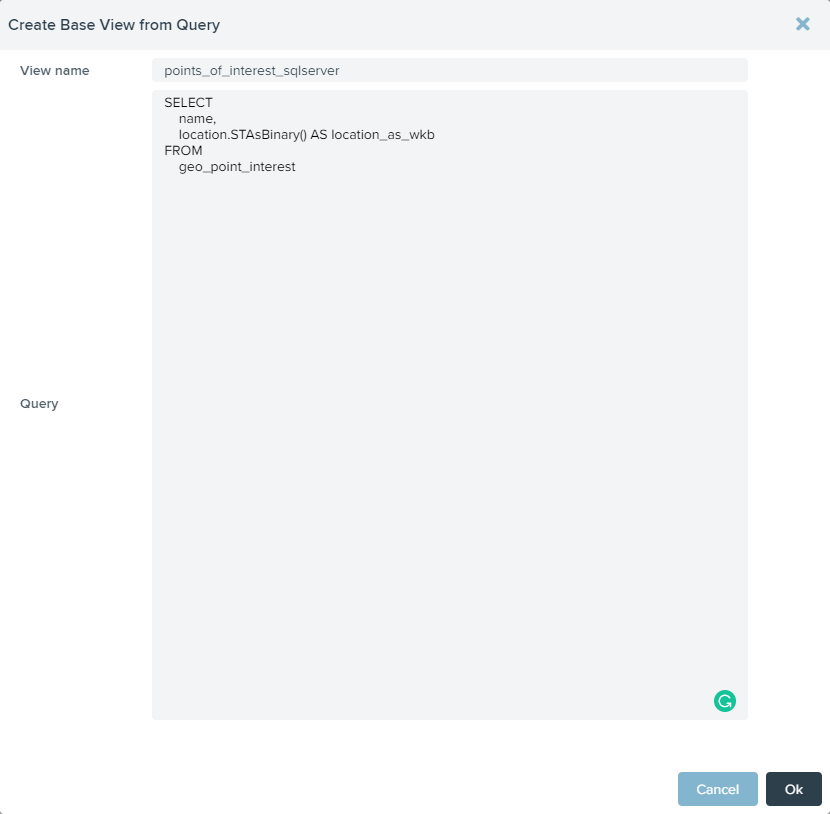Figure 19 Query that obtains the points of interest from Microsoft SQL Server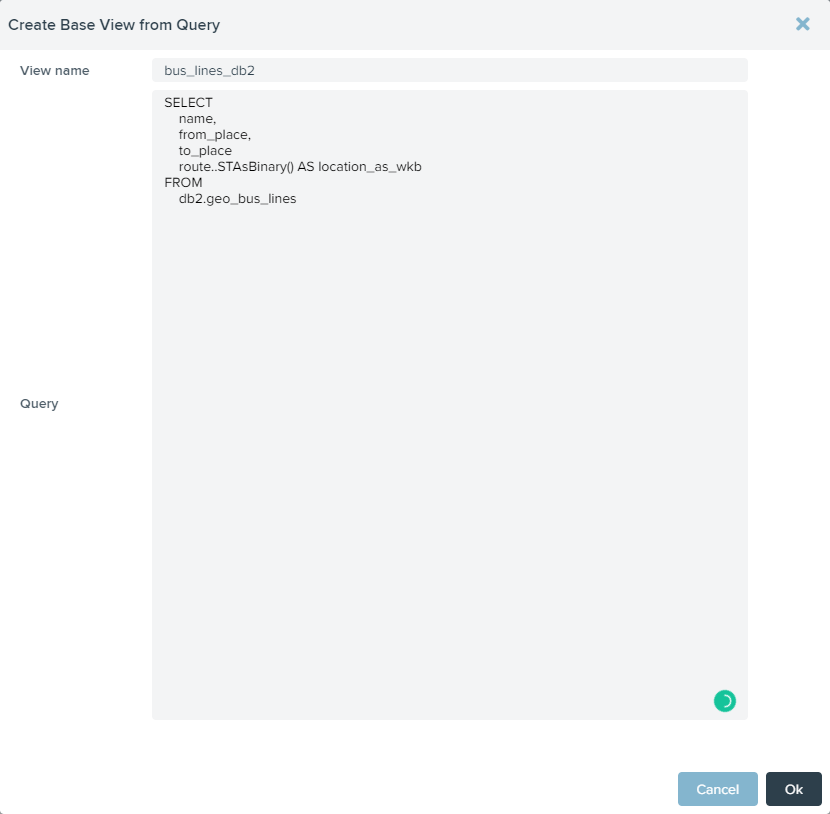Figure 20 Query that obtains the bus lines from IBM DB2

The Figure 21 and the Figure 22 show the views that have been created in Virtual DataPort as a result of the previous queries.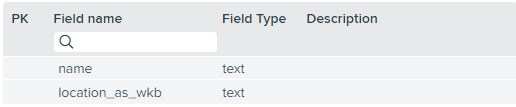Figure 21 View that obtains the points of interest from Microsoft SQL Server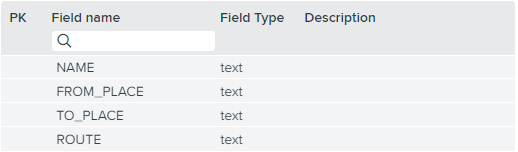Figure 22 View that obtains the bus lines from IBM DB2

Then, we have to create an auxiliary view that adds a new field to the view points_interest_sqlserver. For each point of interest, this new field, called minimum_distance, will contain the distance to the nearest bus line. This information will be used to obtain which is the closest bus line.

We need to calculate the distance from each point of interest to each bus line. To do this, we create a cross join view between the views        points_interest_sqlserver and bus_lines_db2 (see Figure 23). The results are grouped by the fields of the view points_interest_sqlserver because we need to obtain the minimum value of the distances for each point of interest (see Figure 24).

Finally, we calculate the minimum of the distances to each bus line (see Figure 25) and project it along with the fields of the view points_interest_sqlserver (see Figure 26). Note that we use the custom function st_distance defined in Figure 8 to calculate the distance between geometries from different data sources. The result is the view points_interest_with_minimum_distance shown in the Figure 27.Figure 23 Model tab of the auxiliary view points_interest_with_minimum_distance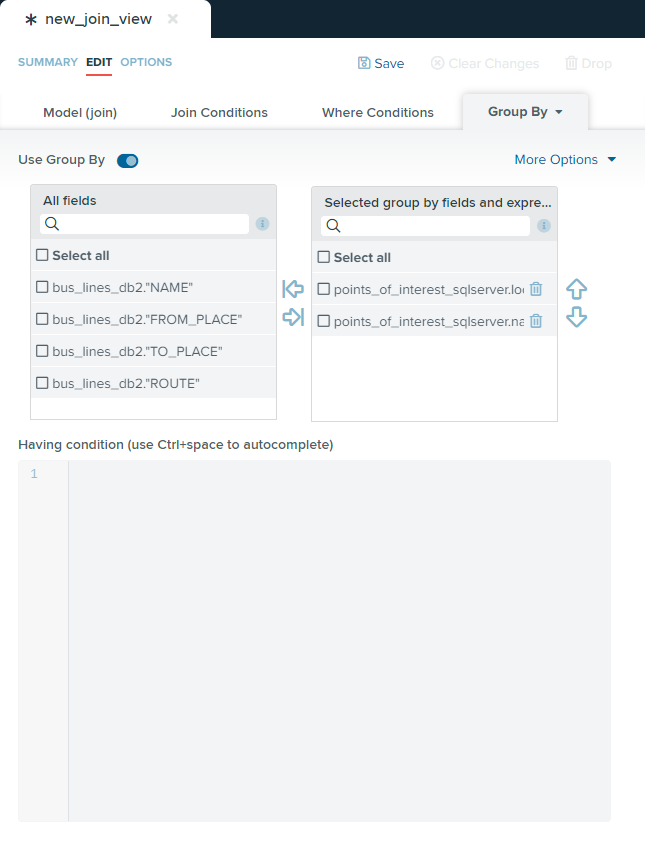Figure 24 Group by tab of the auxiliary view points_interest_with_minimum_distance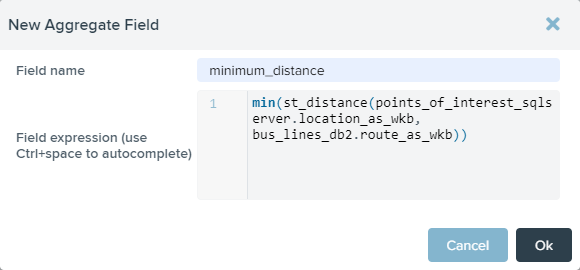Figure 25 New field of the auxiliary view points_interest_with_minimum_distance, which calculates, for each point of interest, the minimum of the distances to each bus line.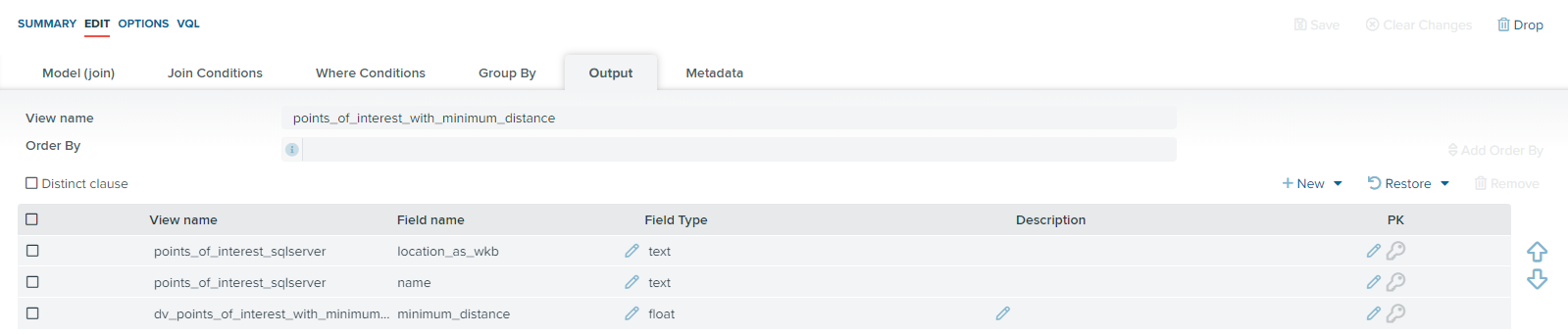Figure 26 Output tab of the auxiliary view points_interest_with_minimum_distance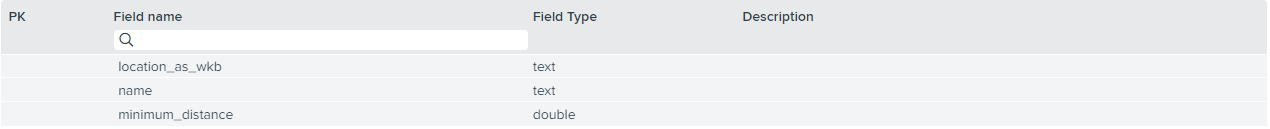Figure 27 View that obtains the points of interest with the distance to the nearest bus line

The query on Figure 28 obtains the closest bus line(s) to each point of interest in the city. Basically, the query is a JOIN between the points of interest and those bus lines whose distance coincides with the minimum distance.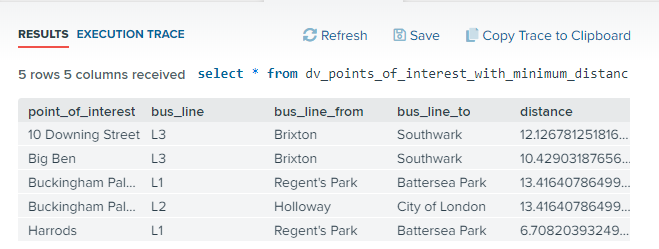Figure 28 Query that retrieves the closest bus line(s) to each point of interest

 For further information, see the section Developing Custom Functions of the Virtual DataPort Developer Guide.

 The Denodo XtraFuncs for VDP library is available to download on the Denodo Connect section of the Denodo Support Site.

 To parse the input expressed as WKT or WKB, we have used the library JTS Topology Suite.

### Featured content

#### DENODO TRAINING

Ready for more? Great! We offer a comprehensive set of training courses, taught by our technical instructors in small, private groups for getting a full, in-depth guided training in the usage of the Denodo Platform. Check out our training courses.